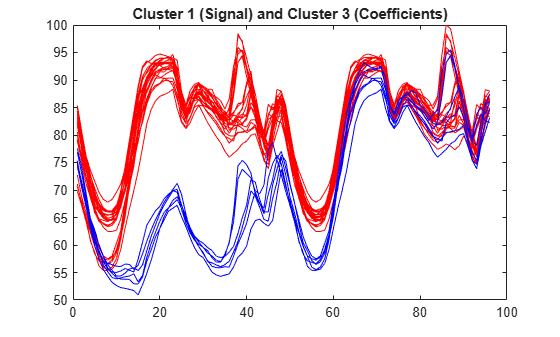# mdwtcluster

Multisignals 1-D clustering

## Syntax

``s = mdwtcluster(x)``
``s = mdwtcluster(___,Name,Value)``

## Description

````s = mdwtcluster(x)` clusters data using hierarchical clustering. The input matrix `x` is decomposed in the row direction using the discrete wavelet transform (DWT) with the Haar wavelet and the maximum allowed level `fix(log2(size(x,2)))`. Note`mdwtcluster` requires Statistics and Machine Learning Toolbox™. ```

example

````s = mdwtcluster(___,Name,Value)` specifies options using name-value pair arguments in addition to the input argument in the previous syntax. For example, `'level',4` specifies the decomposition level. ```

## Examples

collapse all

Load the 1-D multisignal `elecsig10`.

`load elecsig10`

Compute the structure resulting from multisignal clustering.

```lst2clu = {'s','ca1','ca3','ca6'}; S = mdwtcluster(signals,'maxclust',4,'lst2clu',lst2clu)```
```S = struct with fields: IdxCLU: [70x4 double] Incons: [69x4 double] Corr: [0.7920 0.7926 0.7947 0.7631] ```

Retrieve the cluster indices.

`IdxCLU = S.IdxCLU;`

Plot the first and third clusters.

```plot(signals(IdxCLU(:,1)==1,:)','r') hold on plot(signals(IdxCLU(:,1)==3,:)','b') hold off title('Cluster 1 (Signal) and Cluster 3 (Coefficients)')```Check the equality of partitions. Confirm we obtain the same partitions using coefficients of approximation at level 3 instead of the original signals. Much less information is then used.

`equalPART = isequal(IdxCLU(:,1),IdxCLU(:,3))`
```equalPART = logical 1 ```

## Input Arguments

collapse all

Input data, specified as a matrix.

Data Types: `single` | `double` | `int8` | `int16` | `int32` | `int64` | `uint8` | `uint16` | `uint32` | `uint64`

### Name-Value Arguments

Specify optional pairs of arguments as `Name1=Value1,...,NameN=ValueN`, where `Name` is the argument name and `Value` is the corresponding value. Name-value arguments must appear after other arguments, but the order of the pairs does not matter.

Before R2021a, use commas to separate each name and value, and enclose `Name` in quotes.

Example: `s = mdwtcluster(signals,'maxclust',4,'wname','db4')` specifies four clusters and the wavelet `db4`.

Direction of decomposition, specified as `'r'` (row) or `'c'` (column).

Level of DWT decomposition, specified as a positive integer. The default value is `fix(log2(size(x,d)))`, where `d=1` or `d=2`, depending on the `dirDec` value.

Wavelet used for the DWT, specified as a character vector or string scalar. The default value is the Haar wavelet, `'haar'`.

DWT extension mode, specified as a character vector or string scalar. See `dwtmode`.

Distance metric, specified as a character vector, string scalar, or function handle. The default value is `'euclidean'`. See `pdist` (Statistics and Machine Learning Toolbox).

Algorithm for computing the distance between clusters, specified as one of the values in this table.

MethodDescription
`'average'`

Unweighted average distance (UPGMA)

`'centroid'`

Centroid distance (UPGMC), appropriate for Euclidean distances only

`'complete'`

Farthest distance

`'median'`

Weighted center of mass distance (WPGMC), appropriate for Euclidean distances only

`'single'`

Shortest distance

`'ward'`

Inner squared distance (minimum variance algorithm), appropriate for Euclidean distances only

`'weighted'`

Weighted average distance (WPGMA)

See `linkage` (Statistics and Machine Learning Toolbox).

Number of clusters, specified as an integer or vector.

Cell array of character vectors or string vector which contains the list of data to classify. If N is the level of decomposition, the allowed name values for the cells are:

• `'s'` — Signal

• `'aj'` — Approximation at level j

• `'dj'` — Detail at level j

• `'caj'` — Coefficients of approximation at level j

• `'cdj'` — Coefficients of detail at level j

with ```j = 1, …, N```.

The default value is `{'s';'ca1';...;'caN'}` or `["s" "cal" ... "caN"]`.

## Output Arguments

collapse all

The output structure `s` is such that for each partition j:

 `S.Idx(:,j)` Contains the cluster numbers obtained from the hierarchical cluster tree. See `cluster` (Statistics and Machine Learning Toolbox). `S.Incons(:,j)` Contains the inconsistent values of each non-leaf node in the hierarchical cluster tree. See `inconsistent` (Statistics and Machine Learning Toolbox). `S.Corr(j)` Contains the cophenetic correlation coefficients of the partition. See `cophenet` (Statistics and Machine Learning Toolbox).

Note

If `maxclust` is a vector, then `IdxCLU` is a multidimensional array such that `IdxCLU(:,j,k)` contains the cluster numbers obtained from the hierarchical cluster tree for k clusters.

## Version History

Introduced in R2008a